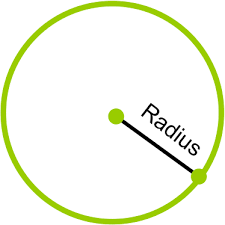Problems
315 - Circle

Circle

Time Limit: 1 sec

The Problem

Suppose there is a Circle. The Radius will be given.

Note : The Area of Circle is pi * (Radius)2

Here pi = 3.1416The Input

Input file contains a series of line. Each line contains a single integer number, the Radius of the Circle, R (R<100). Input is terminated by EOF

The Output

For each line of input print the Area of the Circle rounded to 2 decimal points in a separate line.

Sample Input

10

8

5

Sample Output

314.16

201.06

78.54

Problem Setter: Samia Safa Ahmed